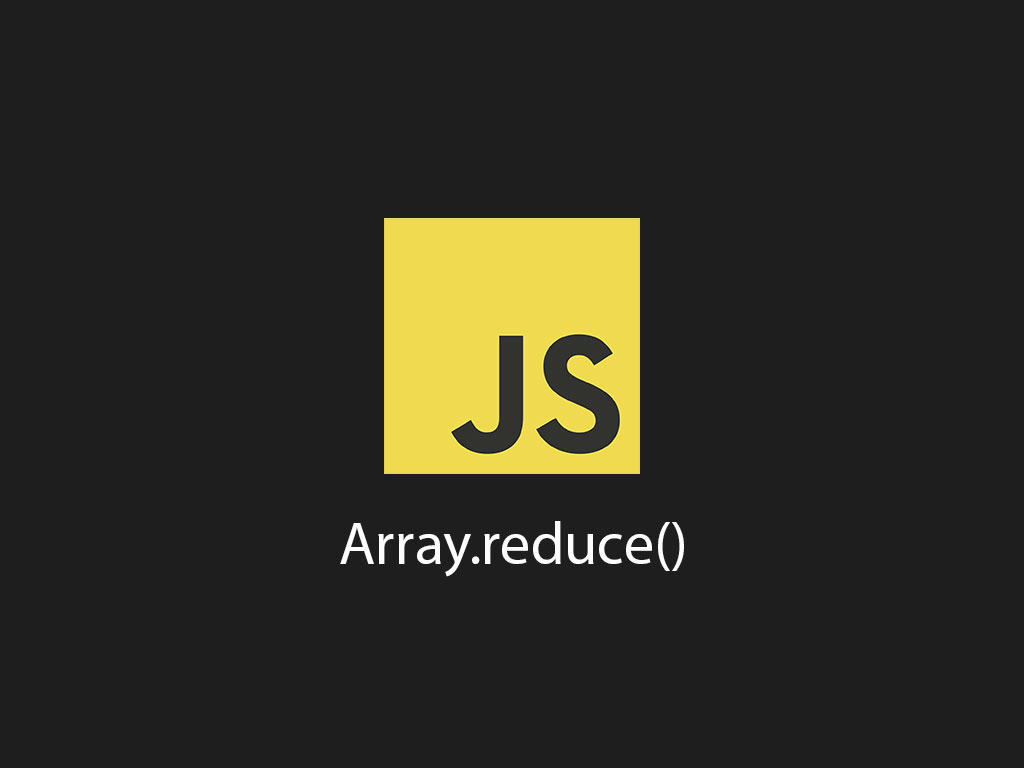# A simple introduction to JavaScript method reducerHave you ever needed to go through an array and sum it into a single value?

I remember the first 4 years of my programming career, I just used a bunch of for loops.

But then I discovered Array.reduce() and that made my JavaScript programming better, and more legible.

``````
const orders = [
{ animal: 'cat', amount: 200 },
{ animal: 'dog', amount: 743 },
{ animal: 'dog', amount: 402 },
{ animal: 'cat', amount: 300 },
];

let totalAmount = 0;
for (let index = 0; index < orders.length; index++) {
totalAmount += orders[index].amount;
}

// Output: 1645
console.log(totalAmount);
``````

This is all find and dandy to find the total amount of all the orders, but we can use JavaScript reduce to clean this code up, and get the same output.

``````
const totalAmount = orders.reduce(function(sum, order) {
return sum + order.amount;
}, 0);
``````

Code breakdown!

Array.reduce() accepts a callback function, and provides 3 variables to you.

• Accumulator - The result or total of the values.
• Current value - Current element value in the array.
• Index - The index of the current element in the array.

Array.reduce() also accepts an initial value as the second parameter. This is an optional parameter.

But if you don't supply an initial value, JavaScript with throw a TypeError. So, it's not really optional. merp.

In the code example above, I'm providing an initial value of 0. I'm indicating that this totalAmount variable will be a number.

And in my callback function, I'm doing a sum of the total sum plus the order amount. Just like the for loop example.

I can go a bit further, and use ES6 arrow function to clean this up.

``````
const totalAmount = orders.reduce((sum, order) => sum + order.amount, 0);
``````

Now it's a 1 liner!

You might be wondering, sure this cleaner, but is it really better?

Array.reduce() is not like other array methods such as map, filter, or sort, but it is part of the higher-order functions, which means you can chain it with other array higher order functions.

Here's what I mean, I want to get the total amount for only dog type animals.

Let's see how this is written in a for loop.

``````
let totalAmount = 0;
for (let index = 0; index < orders.length; index++) {
if ('dog' === orders[index]) {
totalAmount += orders[index].amount;
}
}
``````

Okay, this is still legible, but you can see that this can get messy real quick though. Especially with more complex arrays.

Let's use some of the array higher order functions with Array.reduce() to get the sum now.

``````
const totalAmountForDogs = orders
.filter(order => 'dog' === order.animal)
.reduce((sum, order) => sum + order.amount, 0);
``````

Yes! This is much easier to read.

I'm using the Array.filter() method to only get dogs, and then Array.reduce() to get the total amount price for all dogs.

Here's another example, that shows how to reduce an array into a single object.

I'm going to use Array.reduce() to build a receipt object. This object will show the number of pets being purchased with the total price.

``````
const receipt = orders
.reduce((receipt, order) => {
return {
numberOfPets: receipt.numberOfPets += 1,
total: receipt.total + order.amount
};
}, { numberOfPets: 0, total: 0 });

// { numberOfPets: 2, total: 1145 }
console.log(receipt);
``````

This time I started with an object as the initial value. This object has 2 default properties, numberOfPets and total.

And as the array of orders in iterating, I'm build the receipt inside the Array.reduce() callback function.

## Conclusion

Array.reduce() by itself is not very impressive and it may not save you a whole lot of lines code. But the real beauty of it comes when you can chain it with other array methods.

Use it as much as you can! Happy coding!

I like to tweet about JavaScript and post helpful code snippets. Follow me there if you would like some too!